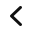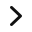# 3 Foolproof Ways to Teach Multiplication for the Best Results

Let me show you the 3 methods I use to conceptually teach multiplication to my third-grade students – and they always work!

The gateway to learning algebra and higher forms of mathematics is multiplication or multiplicative reasoning.  It is critically important to teach multiplication as a type of reasoning using visual models such as equal groupings, arrays, and equal distance jumps on a number line.

I am a big believer in using manipulatives and concrete objects to teach any math concept to elementary school children. That’s why I use manipulatives to teach multiplication.  The math program my district uses comes with many manipulatives including foam tiles.  But, you can use anything:  beans, counters, buttons, etc.

But it is also essential to use the manipulatives with strategies that can also transfer to paper and pencil models.  Though there are many ways to teach multiplication, I have always used 3 foolproof ways that visually show multiplication: equal groups, arrays, and number lines. Why? Not only do they visually show multiplication, but they can easily be transferred to pictorial models.

UPDATE: November 2020

STOP! I wrote another blog post with ideas for teaching multiplication virtually when we switched to distance learning. You can see it HERE!

Wait! Are you introducing multiplication for the first time to your 3rd graders? Then check out this recent post featuring an activity (and FREE download) for introducing multiplication.

## Developing Multiplicative Reasoning

When students begin learning multiplication in second grade by arranging a group of objects into an array and adding by 2s, 3s, 4s, and 5s, we are starting the journey to thinking multiplicatively.

Instead of counting a set of objects to get a total (I counted 4 red crayons and then counted 4 more blue crayons so now I have 8 crayons total), I’m now going to think of 2 sets of 4 crayons (2 sets of 4 is the same as 8). This jump to multiplicative reasoning is part of teaching multiplication. That is why multiplication needs to be visual so students can visualize these equal sets of groups or rows or columns or distances.

## Teach Multiplication with Equal Groups

The first strategy for teaching multiplication is with equal groups.  After all, multiplication is the grouping of equal numbers of objects to more efficiently find a total.  Students must practice forming, identifying, and counting equal groups.  The student’s desktops become whiteboards in my class.

For example, a student gets 12 tiles.  The student forms equal groups with the tiles.  The students will form different combinations. Students can compare the different combinations of equal groups. With 12 tiles, you can form 3 groups of 4 or 4 groups of 3 or 6 groups of 2 or 2 groups of 6 or 1 group of 12 or 12 groups of 1.

Once grouped, students can draw circles around each group and then use skip counting.  Students practice forming equal groups many times until I give them a number that cannot be formed into equal groups such as 13.

When initially teaching multiplication it is important to have students explain why 13 can not be made into equal groups. DISCLAIMER: technically it can because you can divide one tile in half. But third and fourth graders are only learning whole number multiplication at this point.

What you want students to understand and explain is that to think multiplicatively, I need to count equal sets. By grouping objects into equal sets, I become more efficient in my thinking – I’m thinking multiplicatively!

## Teaching Multiplication with Equal Groups using Videos

Here are some videos that illustrate the concept of equal groups.  They can be assigned to the students to watch individually or watch it as a class.  They are kid-friendly and offer good examples and explanations.

If you’re using Google Classroom, you can post them as a resource for students to use at home or in the classroom.  If you use EdPuzzle.com, these videos are excellent for prompting the students with questions so that viewing the video is just not a passive experience.

This particular video teaches multiplication as the ideas of groups, by using real-life examples of “group holders.”

## Teaching Multiplication with Arrays

If students understand equal groups, it’s time to introduce arrays.  Teaching multiplication with arrays is akin to using ten frames to teach addition.  Arrays provide a structured way to see groups. Using arrays makes understanding multiplication clearer because of the rows and columns.

I like to start out a lesson on arrays by asking how groups could be arranged in a way to make them easier to count.  Eventually, someone discovers or builds an array.  Then we have a discussion of why arrays are easier to use.

Just like the practice, we had with forming equal groups, teaching multiplication now involves practicing forming arrays. My students receive multiple opportunities to form rows and columns of equal sets.

You can also show them real-life examples of everyday objects that are group into arrays. Check out this video!

As part of teaching multiplication, arrays are also a useful tool for discovering the Commutative Property of Multiplication.  Just rotate an array 90 degrees, and you have a related fact!  If you have students eventually draw their own arrays, it is a good idea to use graph paper.  Graph paper will help the students keep their arrays from morphing into uncountable blobs!

## More Videos to Demonstrate Using Arrays for Multiplication

This particular video is very useful because it also prompts the students with questions that make it more interactive.

Which kid doesn’t know about MineCraft®!  Keep the motivation going with this MineCraft® themed explanation of arrays.

## Teaching Multiplication with Number Lines

I always use this method last.  Why? Because though it looks straightforward to use, students make many mistakes when using it!  Sometimes students do not count enough spaces to jump or confuse jumps with how many to jump at once.  When using number lines to teach multiplication, the teacher should know that students will have these difficulties so they need to be addressed in the lesson.

I do have number lines that are laminated, and the students put them on a marker board to use. Number lines do not lend themselves very well when using manipulatives.  But by this time, most understand the concept of equal groups which translates into equal distance jumps.

I’ve also thought about using an open number line to multiply as this may lead to less confusion counting the tick marks to jump.  An open number line requires the student to SKIP count by a certain number for each jump.  In contrast, a marked number line requires a student to count the same amount of ticks each time.

When you think of teaching multiplication (and multiplicative reasoning), jumping on an open number line makes more sense. The student has to envision each jump as an equal set or length.

Here are some videos that can be used for a review or for teaching how to use the number line to multiply.

This first one also points out to students common mistakes when using the number line!

Here’s an example of using an open number line.

## Teach the Concept of Multiplication Using a PowerPoint

Once I have established the concept of multiplication using each of these strategies by using manipulatives I want my students to start connecting multiplication to multiplication expressions. These expressions represent equal groups, arrays, and number lines. To help with this, I created a PowerPoint that explicitly explains how to write multiplication equations.

It’s a three-part PowerPoint that teaches equal groups, arrays, and number lines to teach the concept of multiplication.  I also developed printables that come along with the PowerPoint. The printables are used to engage the students with the content of the slide.

The printables help connect the manipulatives to writing multiplication expressions. The PowerPoint engages the students through animation, sound, and printables.  Students also engage with the PowerPoint through embedded questions on each slide.

The Presenter’s Notes for the teacher also guide the teacher through the PowerPoint lessons and provide questions for stimulating mathematical thinking.

Take a look at the full PREVIEW HERE. The preview shows each slide in the PowerPoint and all the printables that are included.

## Self-Checking Multiplication Game!

Do you need your students to practice solving multiplication equations using arrays, equal groups, and number lines? What about practicing solving multiplication word problems? Do you need to have your students practice virtually? Then, check out this newest resource for multiplication: a self-checking Google Slides Multiplication Practice Game!## Don’t Go Yet!

Or register for the newsletter to receive this FREE Guide to Achieving Multiplication Fluency. Get it now by signing up for my newsletter below!

What are your go-to strategies for teaching multiplication?1.